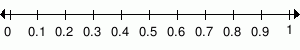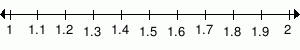MathScore EduFighter is one of the best math games on the Internet today. You can start playing for free!

## Compare Decimals - Sample Math Practice Problems

The math problems below can be generated by MathScore.com, a math practice program for schools and individual families. References to complexity and mode refer to the overall difficulty of the problems as they appear in the main program. In the main program, all problems are automatically graded and the difficulty adapts dynamically based on performance. Answers to these sample questions appear at the bottom of the page. This page does not grade your responses.

See some of our other supported math practice problems.

### Complexity=1, Mode=mod

Use >, <, or = to relate the two numbers.

 1.0.8 --- > < = 0.6 2.1.1 --- > < = 1.7

### Complexity=2, Mode=mod

Use >, <, or = to relate the two numbers.

1.
Ones.TenthsHundredths
1.73
2.00

1.73 2
2.
Ones.TenthsHundredths
1.30
1.97

1.3 1.97

### Complexity=1

Use >, <, or = to relate the two numbers.

 1.   1.9 --- > < = 1.3 2.   3 --- > < = 2.1

### Complexity=2

Use >, <, or = to relate the two numbers.

 1.   3.65 --- > < = 3.46 2.   2.37 --- > < = 2.66

### Complexity=3

Use >, <, or = to relate the two numbers.

 1.   1.924 --- > < = 1.937 2.   1.412 --- > < = 1.243

### Complexity=4

Use >, <, or = to relate the two numbers.

 1.   2.7587 --- > < = 2.2082 2.   0.001 --- > < = 0.0009

### Complexity=1, Mode=mod

Use >, <, or = to relate the two numbers.

10.8 0.6
21.1 1.7

### Complexity=2, Mode=mod

Use >, <, or = to relate the two numbers.

1
Ones.TenthsHundredths
1.73
2.00

1.73 2
2
Ones.TenthsHundredths
1.30
1.97

1.3 1.97

### Complexity=1

Use >, <, or = to relate the two numbers.

11.9 1.3
23 2.1

### Complexity=2

Use >, <, or = to relate the two numbers.

13.65 3.46
22.37 2.66

### Complexity=3

Use >, <, or = to relate the two numbers.

11.924 1.937
21.412 1.243

### Complexity=4

Use >, <, or = to relate the two numbers.